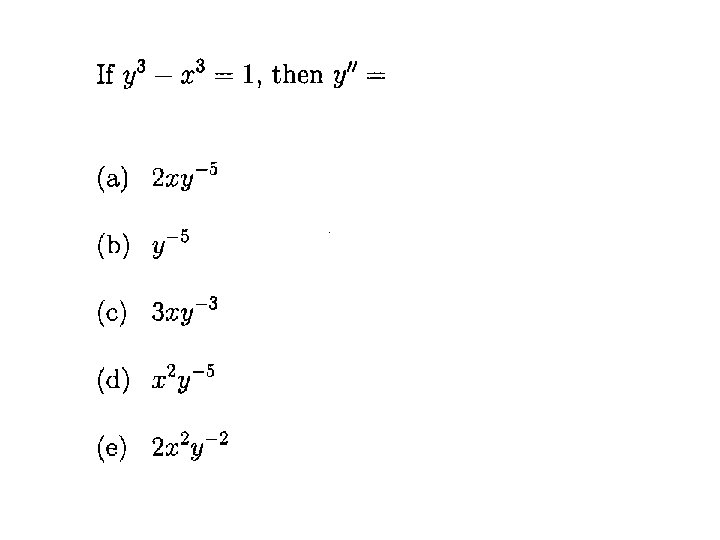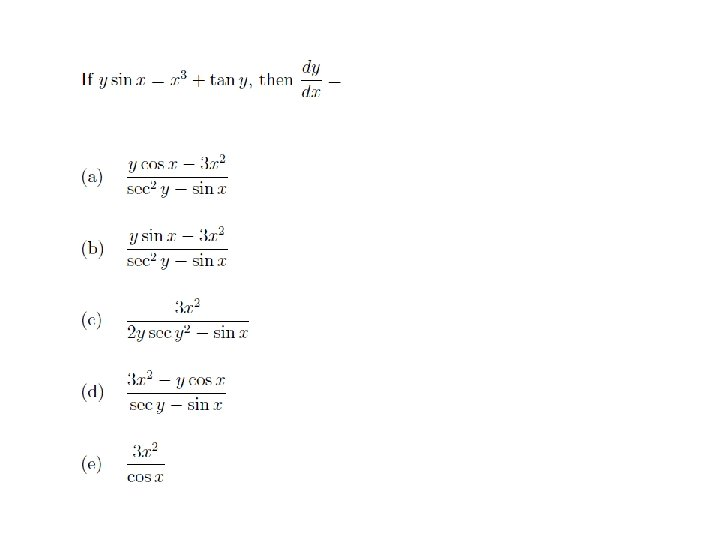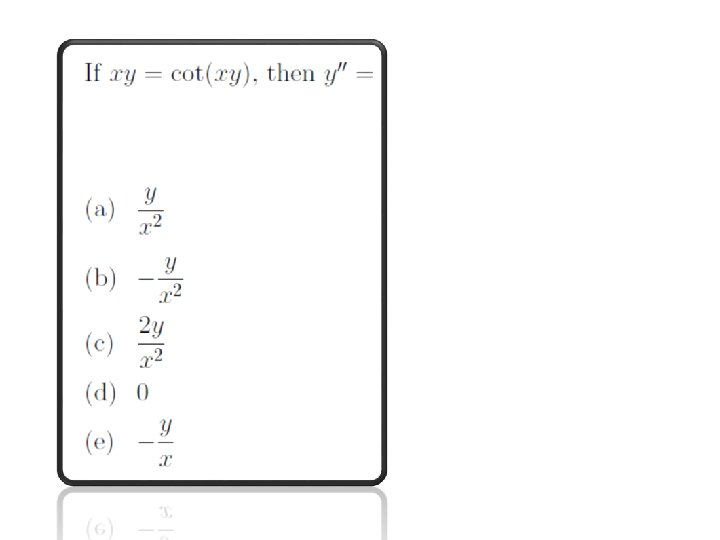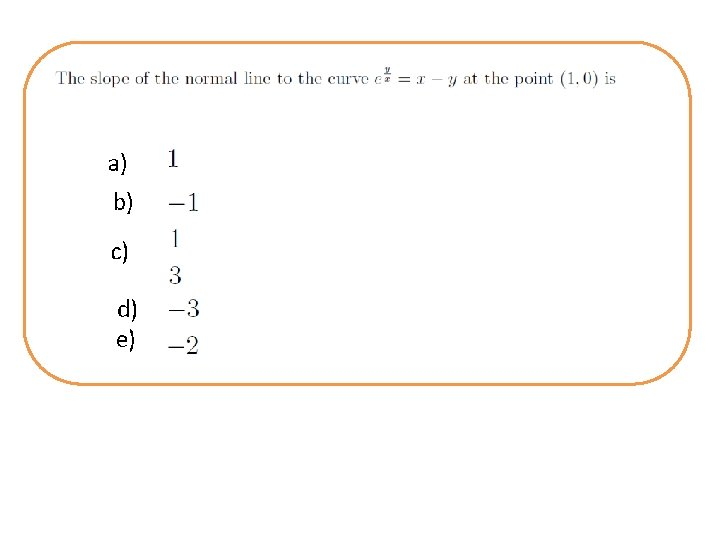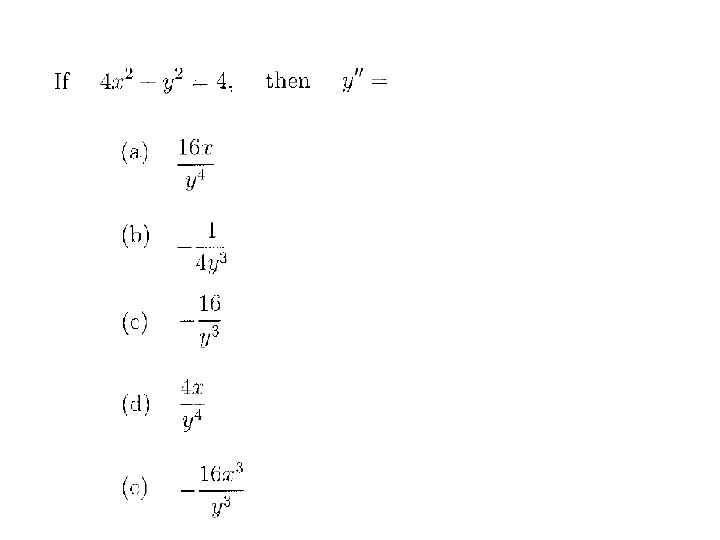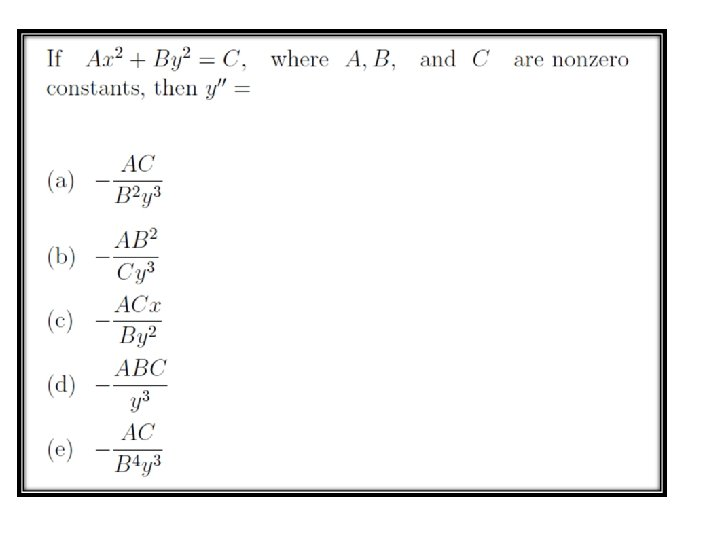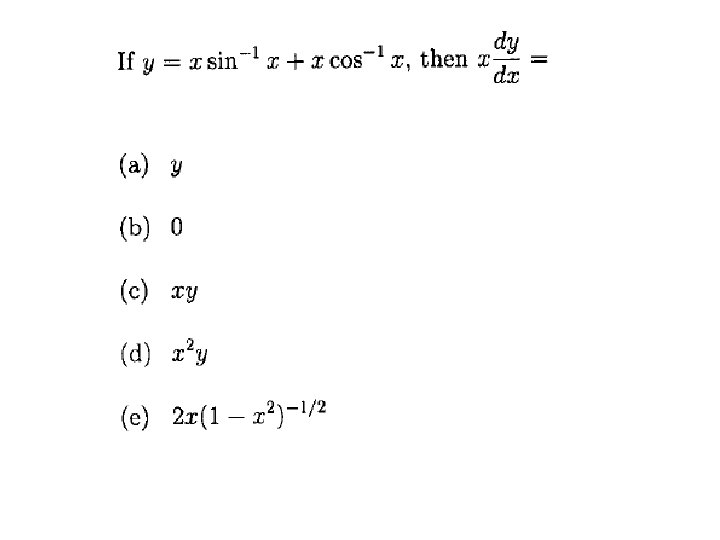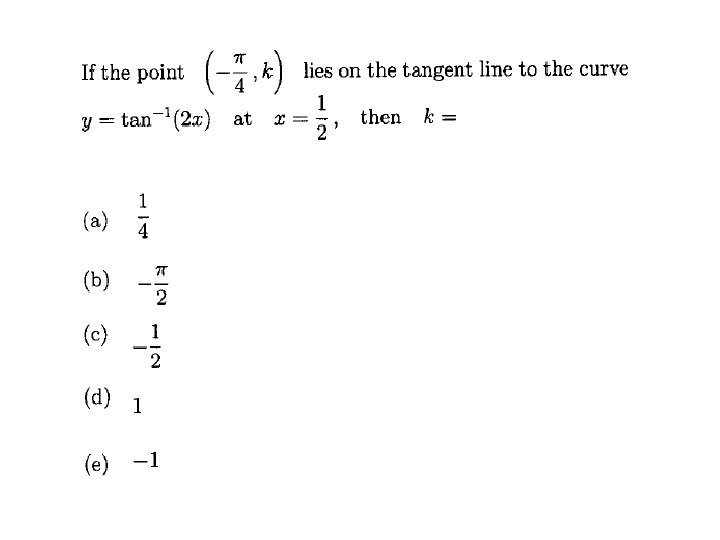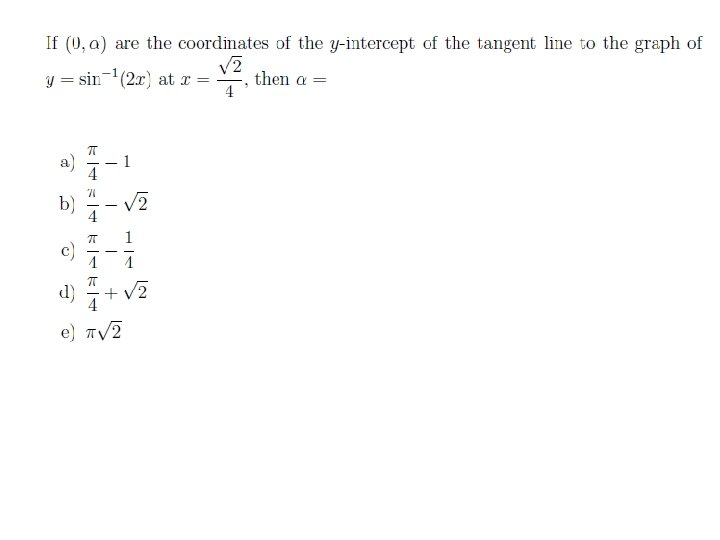# Sec 3 5 IMPLICIT DIFFERENTIATION Example In some

• Slides: 24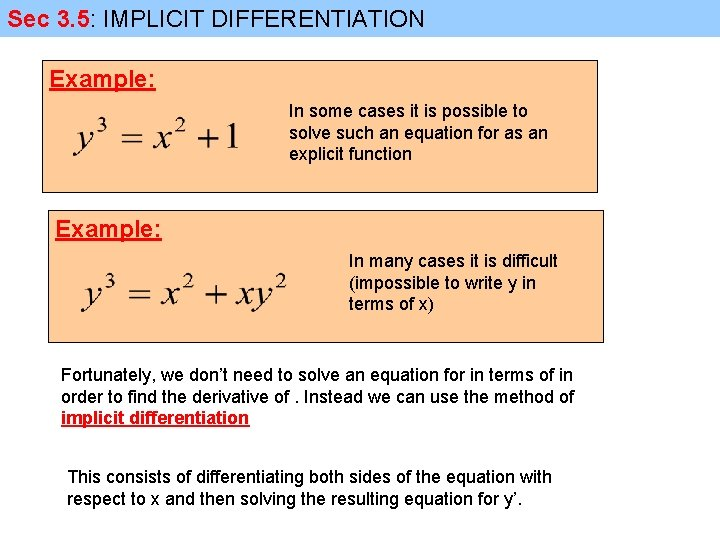Sec 3. 5: IMPLICIT DIFFERENTIATION Example: In some cases it is possible to solve such an equation for as an explicit function Example: In many cases it is difficult (impossible to write y in terms of x) Fortunately, we don’t need to solve an equation for in terms of in order to find the derivative of. Instead we can use the method of implicit differentiation This consists of differentiating both sides of the equation with respect to x and then solving the resulting equation for y’.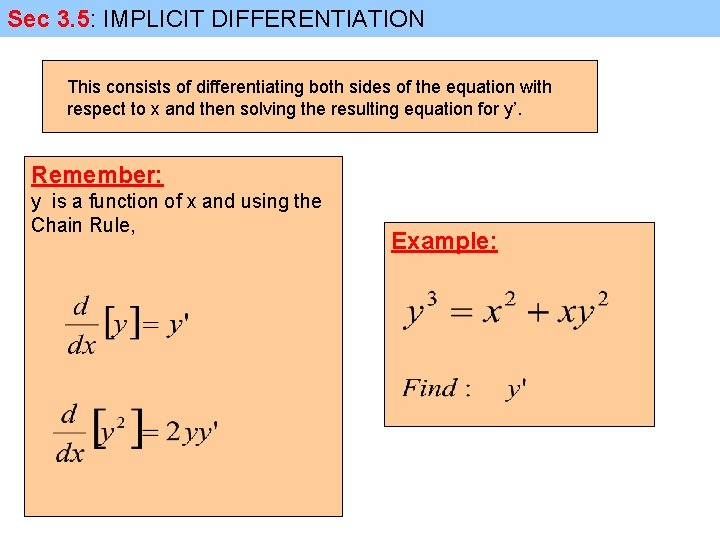Sec 3. 5: IMPLICIT DIFFERENTIATION This consists of differentiating both sides of the equation with respect to x and then solving the resulting equation for y’. Remember: y is a function of x and using the Chain Rule, Example: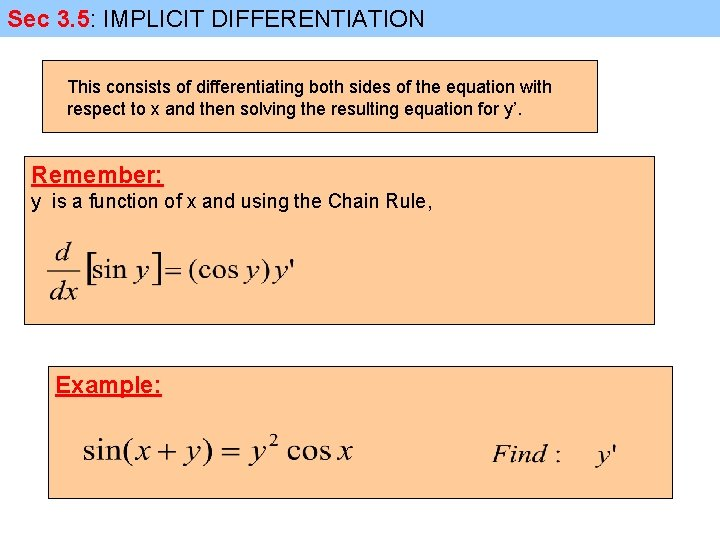Sec 3. 5: IMPLICIT DIFFERENTIATION This consists of differentiating both sides of the equation with respect to x and then solving the resulting equation for y’. Remember: y is a function of x and using the Chain Rule, Example:Sec 3. 5: IMPLICIT DIFFERENTIATION This consists of differentiating both sides of the equation with respect to x and then solving the resulting equation for y’. Remember: y is a function of x and using the Chain Rule,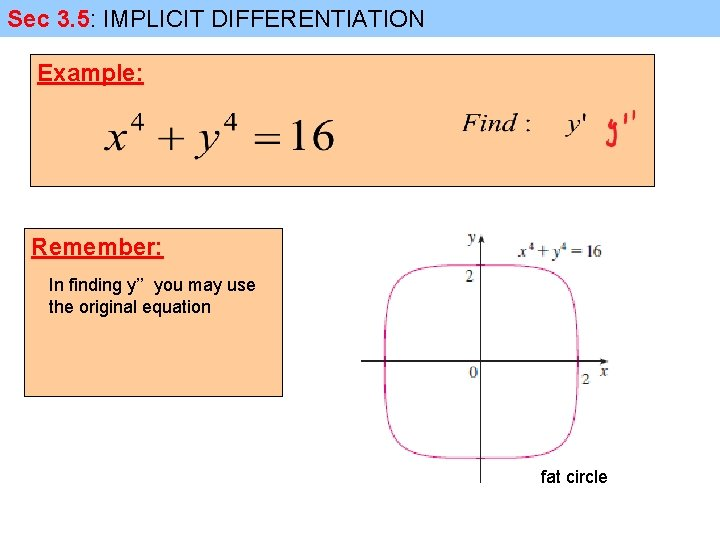Sec 3. 5: IMPLICIT DIFFERENTIATION Example: Remember: In finding y’’ you may use the original equation fat circle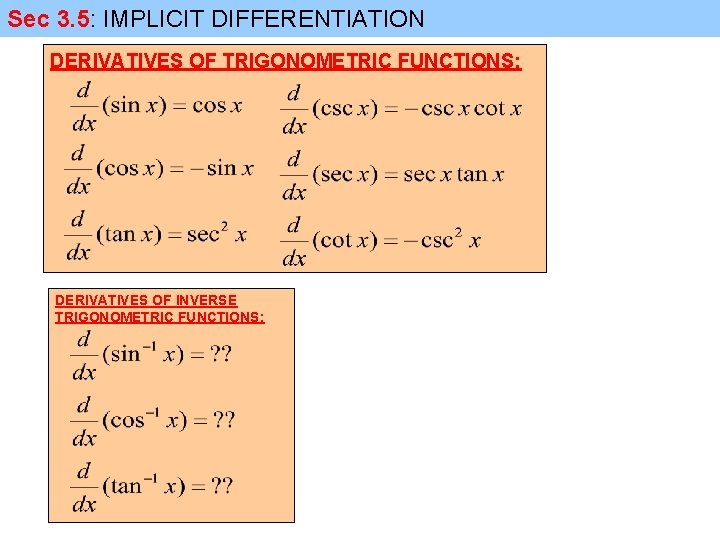Sec 3. 5: IMPLICIT DIFFERENTIATION DERIVATIVES OF TRIGONOMETRIC FUNCTIONS: DERIVATIVES OF INVERSE TRIGONOMETRIC FUNCTIONS: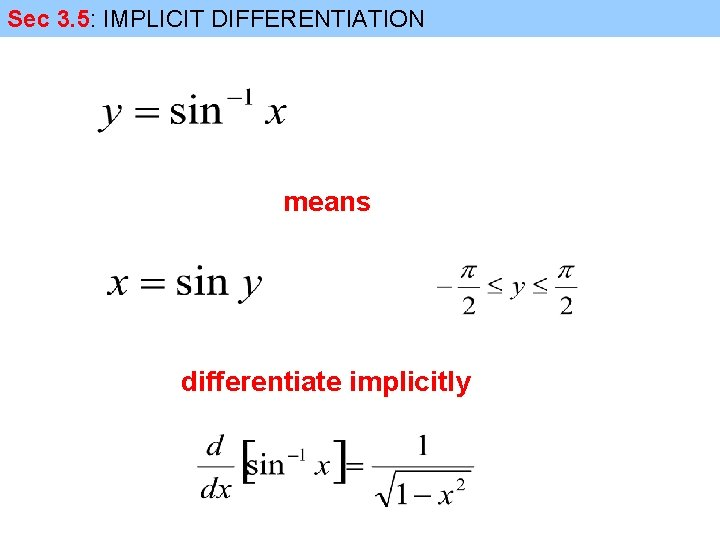Sec 3. 5: IMPLICIT DIFFERENTIATION means differentiate implicitly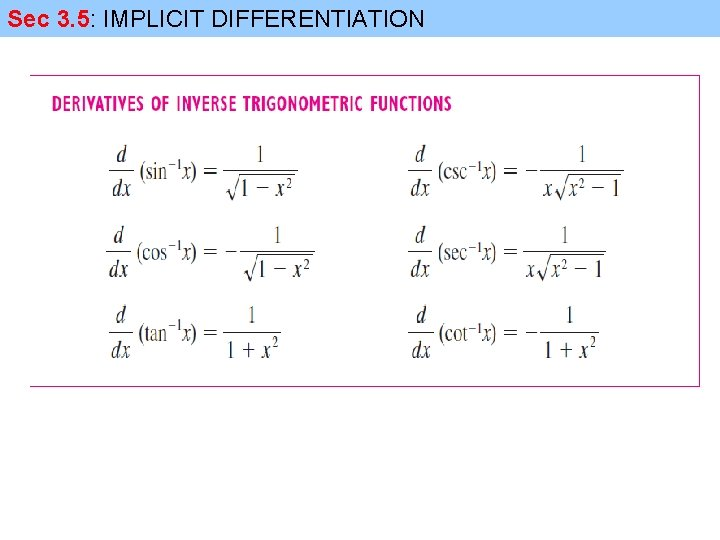Sec 3. 5: IMPLICIT DIFFERENTIATION